## Electric energy and potential

7-8-99

### Potential energy

In discussing gravitational potential energy in PY105, we usually associated it with a single object. An object near the surface of the Earth has a potential energy because of its gravitational interaction with the Earth; potential energy is really not associated with a single object, it comes from an interaction between objects.

Similarly, there is an electric potential energy associated with interacting charges. For each pair of interacting charges, the potential energy is given by:

electric potential energy: PE = k q Q / r

Energy is a scalar, not a vector. To find the total electric potential energy associated with a set of charges, simply add up the energy (which may be positive or negative) associated with each pair of charges.

An object near the surface of the Earth experiences a nearly uniform gravitational field with a magnitude of g; its gravitational potential energy is mgh. A charge in a uniform electric field E has an electric potential energy which is given by qEd, where d is the distance moved along (or opposite to) the direction of the field. If the charge moves in the same direction as the force it experiences, it is losing potential energy; if it moves opposite to the direction of the force, it is gaining potential energy.

The relationship between work, kinetic energy, and potential energy, which was discussed in PY105, still applies:### An example

Two positively-charged balls are tied together by a string. One ball has a mass of 30 g and a charge of 1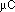; the other has a mass of 40 g and a charge of 2. The distance between them is 5 cm. The ball with the smaller charge has a mass of 30 g; the other ball has a mass of 40 g. Initially they are at rest, but when the string is cut they move apart. When they are a long way away from each other, how fast are they going?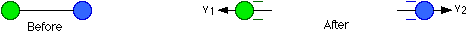Let's start by looking at energy. No external forces act on this system of two charges, so the energy must be conserved. To start with all the energy is potential energy; this will be converted into kinetic energy.

Energy at the start : KE = 0
PE = k q Q / r = (8.99 x 109) (1 x 10-6) (2 x 10-6) / 0.05 = 0.3596 J

When the balls are very far apart, the r in the equation for potential energy will be large, making the potential energy negligibly small.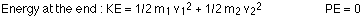Energy is conserved, so the kinetic energy at the end is equal to the potential energy at the start: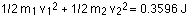The masses are known, but the two velocities are not. To solve for the velocities, we need another relationship between them. Because no external forces act on the system, momentum will also be conserved. Before the string is cut, the momentum is zero, so the momentum has to be zero all the way along. The momentum of one ball must be equal and opposite to the momentum of the other, so: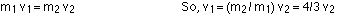Plugging this into the energy equation gives: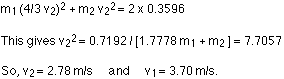### Electric potential

Electric potential is more commonly known as voltage. The potential at a point a distance r from a charge Q is given by:

V = k Q / r

Potential plays the same role for charge that pressure does for fluids. If there is a pressure difference between two ends of a pipe filled with fluid, the fluid will flow from the high pressure end towards the lower pressure end. Charges respond to differences in potential in a similar way.

Electric potential is a measure of the potential energy per unit charge. If you know the potential at a point, and you then place a charge at that point, the potential energy associated with that charge in that potential is simply the charge multiplied by the potential. Electric potential, like potential energy, is a scalar, not a vector.

connection between potential and potential energy: V = PE / q

Equipotential lines are connected lines of the same potential. These often appear on field line diagrams. Equipotential lines are always perpendicular to field lines, and therefore perpendicular to the force experienced by a charge in the field. If a charge moves along an equipotential line, no work is done; if a charge moves between equipotential lines, work is done.

Field lines and equipotential lines for a point charge, and for a constant field between two charged plates, are shown below: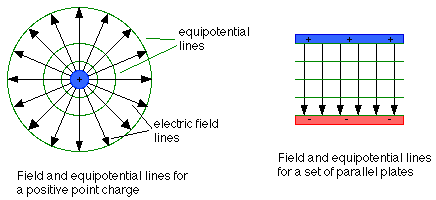### An example : Ionization energy of the electron in a hydrogen atom

In the Bohr model of a hydrogen atom, the electron, if it is in the ground state, orbits the proton at a distance of r = 5.29 x 10-11 m. Note that the Bohr model, the idea of electrons as tiny balls orbiting the nucleus, is not a very good model of the atom. A better picture is one in which the electron is spread out around the nucleus in a cloud of varying density; however, the Bohr model does give the right answer for the ionization energy, the energy required to remove the electron from the atom.

The total energy is the sum of the electron's kinetic energy and the potential energy coming from the electron-proton interaction.

The kinetic energy is given by KE = 1/2 mv2.

This can be found by analyzing the force on the electron. This force is the Coulomb force; because the electron travels in a circular orbit, the acceleration will be the centripetal acceleration: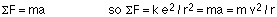Note that the negative sign coming from the charge on the electron has been incorporated into the direction of the force in the equation above.

This gives m v2 = k e2 / r, so the kinetic energy is KE = 1/2 k e2 / r.

The potential energy, on the other hand, is PE = - k e2 / r. Note that the potential energy is twice as big as the kinetic energy, but negative. This relationship between the kinetic and potential energies is valid not just for electrons orbiting protons, but also in gravitational situations, such as a satellite orbiting the Earth.

The total energy is:
KE + PE = -1/2 ke2 / r = - 1/2 (8.99 x 109)(1.60 x 10-19) / 5.29 x 10-11

This works out to -2.18 x 10-18 J. This is usually stated in energy units of electron volts (eV). An eV is 1.60 x 10-19 J, so dividing by this gives an energy of -13.6 eV. To remove the electron from the atom, 13.6 eV must be put in; 13.6 eV is thus the ionization energy of a ground-state electron in hydrogen.